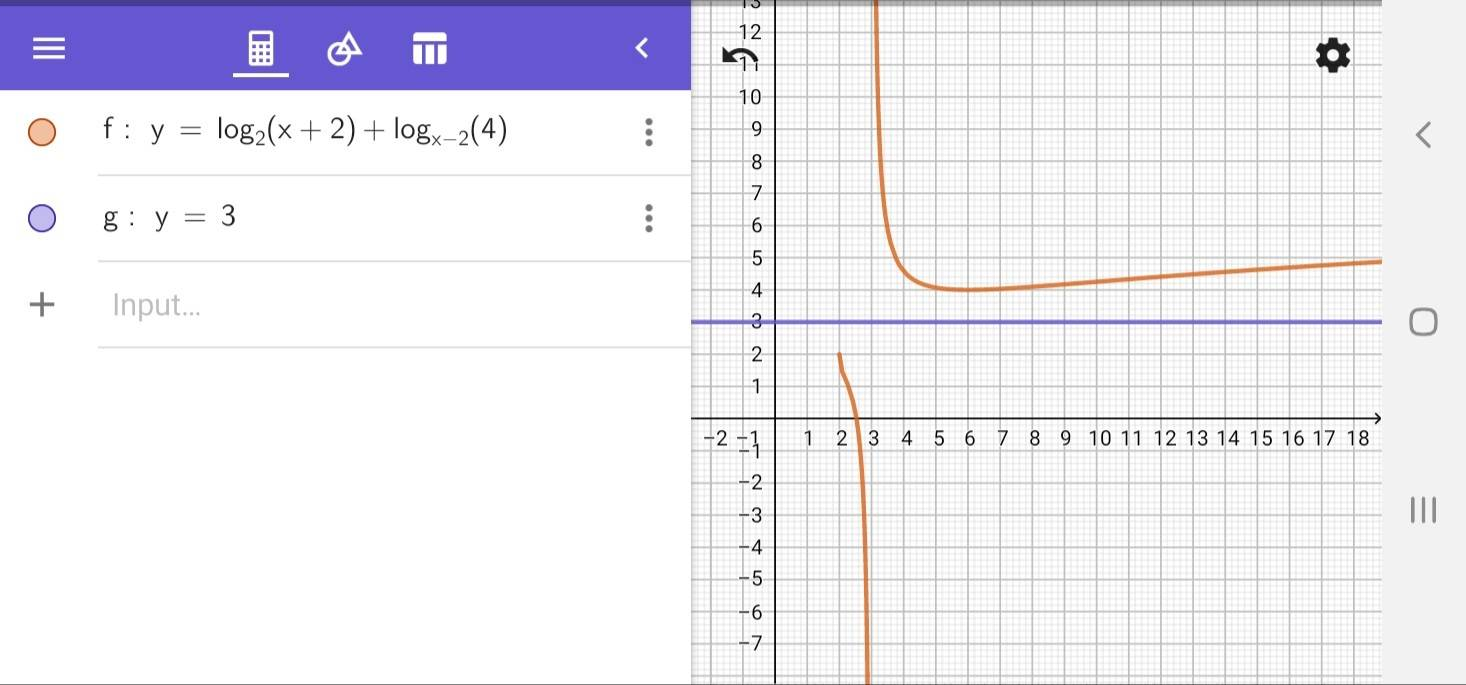• MHB
Monoxdifly
MHB
A friend asked me how to solve this question:
$$\displaystyle log_2(x+2)+log_{(x-2)}4=3$$
I said I had no idea because one is x + 2 and the other one is x - 2. If both are x + 2 or x - 2, I can do it. He said that if that's the case, even at his level he could solve it. This is what I've done so far regarding the question.
$$\displaystyle log_2(x+2)+log_{(x-2)}4=3$$
$$\displaystyle \frac{log(x+2)}{log2}+\frac{log4}{log(x-2)}=3$$
$$\displaystyle \frac{log(x+2)log(x-2)+log4log2}{log2log(x-2)}=3$$
$$\displaystyle log(x+2)log(x-2)+2log^22=3log2log(x-2)$$
$$\displaystyle log(x+2)log(x-2)-3log2log(x-2)=-2log^22$$
$$\displaystyle log(x-2)(log(x+2)-3log2)=-2log^22$$
What should I do from here? Or did I make some mistakes?

jonah1
Beer hangover induced idea follows.Monoxdifly
MHB
So, no x fulfills the equation, right?

skeeter
So, no x fulfills the equation, right?

That's what the graph says ...

Monoxdifly
MHB
Okay, thanks guys.